# 基于偏微分方程解的高温作业服装设计Design of High Temperature Work Clothing Based on Partial Differential Equation Solution

• 全文下载: PDF(1804KB)    PP.464-472   DOI: 10.12677/AAM.2019.83052
• 下载量: 364  浏览量: 1,170   科研立项经费支持

Taking the law of conservation of heat, Fourier heat conduction law, heat formula as the theoretical basis, based on the ambient temperature and body temperature constant, and special clothing material homogeneity assumption, transfer heat in high temperature operation of clothing each layer is simplified to be completely in the adiabatic long pole conduction along the flank. In this way, the heat conduction model of each layer of the high-temperature operating garment is established under the high-temperature operating environment, and then the corresponding optimization design model of layer II and IV thickness of the high-temperature operating garment is constructed according to different design conditions. The genetic algorithm solver provided by MATLAB was used to solve the model.

1. 引言

1) 专用服装材料的密度、比热、热传导率等物理参数已知条件下(见全国大学生数学建模竞赛附件1)，对环境温度为75℃、II层厚度为6 mm、IV层厚度为5 mm、工作时间为90分钟的情形开展实验，测量得到假人皮肤外侧的温度(见全国大学生数学建模竞赛附件2)。建立数学模型，计算专用服装的温度分布。

2) 当环境温度为65℃、IV层的厚度为5.5 mm时，确定II层的最优厚度，确保工作60分钟时，假人皮肤外侧温度不超过47℃，且超过44℃的时间不超过5分钟。

3) 当环境温度为80时，确定II层和IV层的最优厚度，确保工作30分钟时，假人皮肤外侧温度不超过47℃，且超过44℃的时间不超过5分钟。

2. 模型假设

1) 假设专用服装材料I、II、III各层材料的密度、比热、热传导率等物理参数均匀同质，并且不随时间和温度的改变而改变。

2) 本文不考虑专用服装材料I、II、III各层材料的热胀冷缩效应，即I、II、III各层材料的厚度不随温度的改变而改变。

3) 辐射只有在高温下才能成为主要的传热方式(本文的最高温度80℃，远远小于500℃)，对流需要较大的空间(第IV层空隙封闭狭窄)，因此热的传导是各层材料间的热量的传递主要方式，本文不考虑热的辐射传递和对流传递。

4) 假设服装I层外侧的环境温度均匀一致，并且恒定不变。

5) 假设身着高温作业专用服装的假人体内温度为37℃，并且恒定不变，但是体内温度不等同皮肤温度，我们认为温度变化的皮肤到温度恒定的体内存在一个热传导的过程，其间存在一定的介质，本文后面将对这里提到的介质作进一步的假设。

6) 假设专用服装的各层材料的初始温度相同。

7) 假设附件提供的物理参数和测量皮肤外侧的温度是准确的，并且认为皮肤外侧的温度即为第IV层内侧的温度。

3. 符号说明

4. 模型的建立

4.1. 温度分布模型的建立

$\frac{\partial T}{\partial t}=k\frac{{\partial }^{2}T}{\partial {x}^{2}},k>0$

$T\left(x,0\right)={T}_{0}$

$T\left(l,t\right)=f\left(t\right)$

$T\left(0,t\right)=75$

4.2. 第II层厚度的优化模型的建立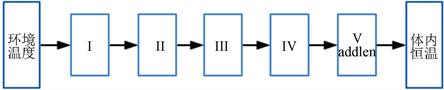Figure 1. Schematic diagram of the five-layer heat conduction model

$\underset{\begin{array}{l}T\left({L}_{4},3300\right)\le 44\\ T\left({L}_{4},3600\right)\le 47\end{array}}{\mathrm{min}}{l}_{2}$

1) 方程：

$\frac{\partial T}{\partial t}=k\frac{{\partial }^{2}T}{\partial {x}^{2}},k>0$

2) 初值条件：

$T\left(x,0\right)={T}_{0}$

3) 边界条件：

$T\left(0,t\right)=65$ , $T\left(L,t\right)=37$

4.3. II层和IV层的最优厚度模型的建立

$\underset{\begin{array}{l}T\left({L}_{4},3300\right)\le 44\\ T\left({L}_{4},3600\right)\le 47\end{array}}{\mathrm{min}}{l}_{2}+{l}_{4}$

$T\left({L}_{4},3300\right)$$T\left({L}_{4},3600\right)$ 由模型2模拟计算(其边界条件： $T\left(0,t\right)=80$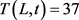)，热扩散率为 ${K}_{5}=0.895$ ，长度为 ${l}_{5}=15.7$ )。

5. 模型求解

5.1. 模型1的求解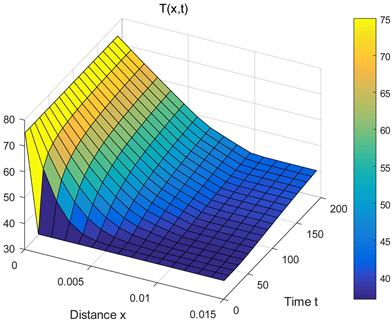Figure 2. Temperature distribution of special clothing for high-temperature operation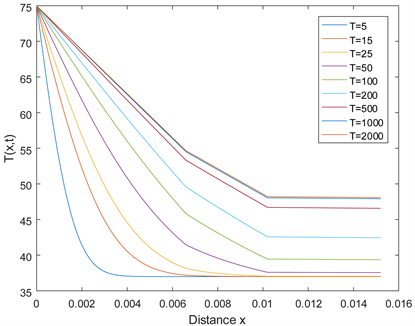Figure 3. Temperature distribution of special clothing for high-temperature operation at a specific time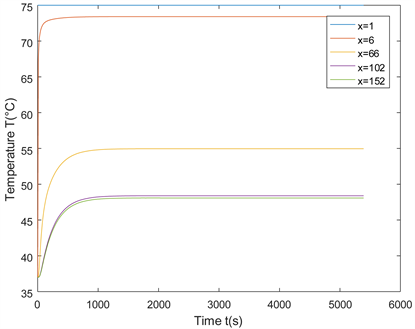Figure 4. Temperature variation of special clothing for high-temperature operation at a specific location

5.2. 模型2的求解

$\underset{{k}_{5},{l}_{5}}{\mathrm{min}}\left(\underset{t=1:5400}{\sum }{\left(T\left({L}_{4},t\right)-Temp\left(t\right)\right)}^{2}\right)$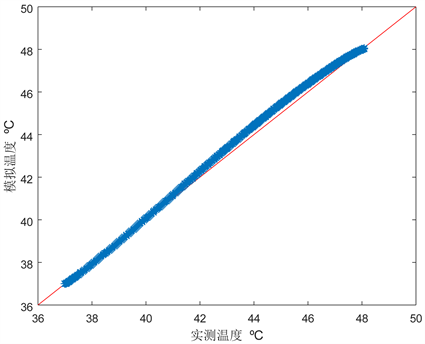Figure 5. The comparison between the calculated skin temperature and the measured temperature at ${k}_{5}=0.895$ ${l}_{5}=15.7$Table 1. The solution of problem two

5.3. 模型3的求解Figure 6. Simulation of temperature change inside layer IV

6. 模型的检验

6.1. 传导方程的检验

6.2. IV层内侧热传递模型的检验

7. 模型的改进和应用

1) 在本文模型的基础上对安全性进行实际检验，确定符合安全性要求“皮肤到温度恒定的体内的热传导的过程，简化看作热在长度一定的均匀介质中的传导过程”的参数确定方案、而不是以拟合程度最佳为依据；

2) 优化模拟程序，提高程序的运行速度和智能化程度。

NOTES

*通讯作者。

  贾海峰. 一维热传导方程的推导[J]. 科技信息, 2013(2): 159.  徐建良, 汤炳书. 一维热传导方程的数值解[J]. 淮阴师范学院学报(自然科学版), 2004(3): 210-214.  卢琳珍, 徐定华, 徐映红. 应用三层热防护服热传递改进模型的皮肤烧伤度预测[J]. 纺织学报, 2018, 39(1): 111-118, 125.  周远, 徐映红, 徐定华. 结合粒子群算法的一类双层纺织材料厚度设计反问题[J]. 纺织学报, 2013, 34(6): 40-45.  徐定华, 葛美宝. 一类纺织材料热湿传递模型的数值解法[J]. 江西科学, 2010, 28(5): 578-582.  Skeel, R.D. and Berzins, M. (1990) A Method for the Spatial Discretization of Parabolic Equations in One Space Variable. SIAM Journal on Scientific and Statistical Computing, 11, 1-32. https://doi.org/10.1137/0911001  李萍, 张磊, 王垚廷. 基于Matlab的偏微分方程数值计算[J]. 齐鲁工业大学学报(自然科学版), 2017, 31(4): 39-43.  黄作英, 阙沛文, 陈亮. PDE工具箱实现偏微分方程的有限元求解[J]. 计算机仿真, 2006(9): 100-102, 115.  李灿, 高彦栋, 黄素逸. 热传导问题的MATLAB数值计算[J]. 华中科技大学学报(自然科学版), 2002(9): 91-93.  初永丽. 一种GA算法的改进及其实现[J]. 微计算机信息, 2006(7): 128-129, 155.  高见文, 葛卫丽, 郭程. 基于粒子群的混合智能优化算法收敛性分析[J]. 电子世界, 2016(20): 98-99.  王晓华, 杨娜. 基于遗传算法的参数优化估算模型[J]. 电子世界, 2012(24): 118-119.